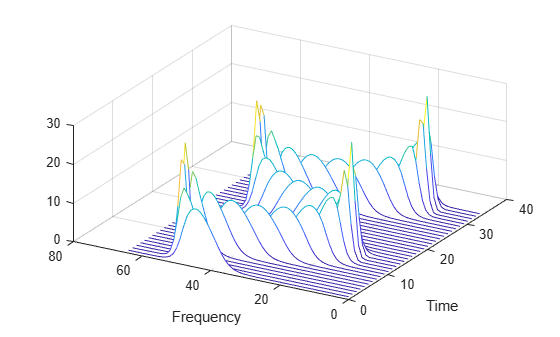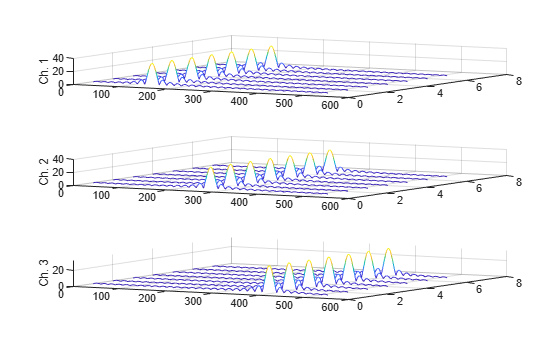# stftLayer

Short-time Fourier transform layer

Since R2021b

## Description

An STFT layer computes the short-time Fourier transform of the input. Use of this layer requires Deep Learning Toolbox™.

## Creation

### Syntax

``layer = stftLayer``
``layer = stftLayer(Name=Value)``

### Description

example

````layer = stftLayer` creates a Short-Time Fourier Transform (STFT) layer. The input to `stftLayer` must be a `dlarray` (Deep Learning Toolbox) object in `"CBT"` format with a size along the time dimension greater than the length of `Window`. `stftLayer` formats the output as `"SCBT"`. For more information, see Layer Output Format.```

example

````layer = stftLayer(Name=Value)` sets properties using one or more name-value arguments. You can specify the analysis window and the number of overlapped samples, among others.```

## Properties

expand all

### STFT

Analysis window used to compute the STFT, specified as a vector with two or more elements.

Example: `(1-cos(2*pi*(0:127)'/127))/2` and `hann(128)` both specify a Hann window of length 128.

Data Types: `double` | `single`

Number of overlapped samples, specified as a positive integer strictly smaller than the length of `Window`.

The stride between consecutive windows is the difference between the window length and the number of overlapped samples.

Data Types: `double` | `single`

Number of frequency points used to compute the discrete Fourier transform, specified as a positive integer greater than or equal to the window length. If not specified, this argument defaults to the length of the window.

Data Types: `double` | `single`

Layer transform mode, specified as one of these:

• `"mag"` — STFT magnitude

• `"squaremag"` — STFT squared magnitude

• `"logmag"` — Natural logarithm of the STFT magnitude

• `"logsquaremag"` — Natural logarithm of the STFT squared magnitude

• `"realimag"` — Real and imaginary parts of the STFT, concatenated along the channel dimension

Data Types: `char` | `string`

### Layer

Multiplier for weight learning rate, specified as a nonnegative scalar. If not specified, this property defaults to zero, resulting in weights that do not update with training. You can also set this property using the `setLearnRateFactor` (Deep Learning Toolbox) function.

Data Types: `double` | `single`

Layer name, specified as a character vector or a string scalar. For `Layer` array input, the `trainnet` (Deep Learning Toolbox), `trainNetwork` (Deep Learning Toolbox), `assembleNetwork` (Deep Learning Toolbox), `layerGraph` (Deep Learning Toolbox), and `dlnetwork` (Deep Learning Toolbox) functions automatically assign names to layers with the name `""`.

The `stftLayer` object stores this property as a character vector.

Data Types: `char` | `string`

Number of inputs to the layer, returned as `1`. This layer accepts a single input only.

Data Types: `double`

Input names, returned as `{'in'}`. This layer accepts a single input only.

Data Types: `cell`

Number of outputs from the layer, returned as `1`. This layer has a single output only.

Data Types: `double`

Output names, returned as `{'out'}`. This layer has a single output only.

Data Types: `cell`

## Examples

collapse all

Generate a signal sampled at 600 Hz for 2 seconds. The signal consists of a chirp with sinusoidally varying frequency content. Store the signal in a deep learning array with `"CTB"` format.

```fs = 6e2; x = vco(sin(2*pi*(0:1/fs:2)),[0.1 0.4]*fs,fs); dlx = dlarray(x,"CTB");```

Create a short-time Fourier transform layer with default properties. Create a `dlnetwork` object consisting of a sequence input layer and the short-time Fourier transform layer. Specify a minimum sequence length of 128 samples. Run the signal through the `predict` method of the network.

```ftl = stftLayer; dlnet = dlnetwork([sequenceInputLayer(1,MinLength=128) ftl]); netout = predict(dlnet,dlx);```

Convert the network output to a numeric array. Use the `squeeze` function to remove the length-1 channel and batch dimensions. Plot the magnitude of the STFT. The first dimension of the array corresponds to frequency and the second to time.

```q = extractdata(netout); waterfall(squeeze(q)') set(gca,XDir="reverse",View=[30 45]) xlabel("Frequency") ylabel("Time")```Generate a 3 × 160 (× 1) array containing one batch of a three-channel, 160-sample sinusoidal signal. The normalized sinusoid frequencies are π/4 rad/sample, π/2 rad/sample, and 3π/4 rad/sample. Save the signal as a `dlarray`, specifying the dimensions in order. `dlarray` permutes the array dimensions to the `"CBT"` shape expected by a deep learning network.

```nch = 3; N = 160; x = dlarray(cos(pi.*(1:nch)'/4*(0:N-1)),"CTB");```

Create a short-time Fourier transform layer that can be used with the sinusoid. Specify a 64-sample rectangular window, 48 samples of overlap between adjoining windows, and 1024 DFT points. By default, the layer outputs the magnitude of the STFT.

```stfl = stftLayer(Window=rectwin(64), ... OverlapLength=48, ... FFTLength=1024);```

Create a two-layer `dlnetwork` object containing a sequence input layer and the STFT layer you just created. Treat each channel of the sinusoid as a feature. Specify the signal length as the minimum sequence length for the input layer.

```layers = [sequenceInputLayer(nch,MinLength=N) stfl]; dlnet = dlnetwork(layers);```

Run the sinusoid through the `forward` method of the network.

`dataout = forward(dlnet,x);`

Convert the network output to a numeric array. Use the `squeeze` function to collapse the size-1 batch dimension. Permute the channel and time dimensions so that each array page contains a two-dimensional spectrogram. Plot the STFT magnitude separately for each channel in a waterfall plot.

```q = squeeze(extractdata(dataout)); q = permute(q,[1 3 2]); for kj = 1:nch subplot(nch,1,kj) waterfall(q(:,:,kj)') view(30,45) zlabel("Ch. "+string(kj)) end```expand all

## Version History

Introduced in R2021b

expand all